Thank you for visiting nature.com. You are using a browser version with limited support for CSS. To obtain the best experience, we recommend you use a more up to date browser (or turn off compatibility mode in Internet Explorer). In the meantime, to ensure continued support, we are displaying the site without styles and JavaScript.

# Entanglement percolation in quantum networks

## Abstract

Quantum networks are composed of nodes that can send and receive quantum states by exchanging photons1. Their goal is to facilitate quantum communication between any nodes, something that can be used to send secret messages in a secure way2,3, and to communicate more efficiently than in classical networks4. These goals can be achieved, for instance, via teleportation5. Here we show that the design of efficient quantum-communication protocols in quantum networks involves intriguing quantum phenomena, depending both on the way the nodes are connected and on the entanglement between them. These phenomena can be used to design protocols that overcome the exponential decrease of signals with the number of nodes. We relate the problem of establishing maximally entangled states between nodes to classical percolation in statistical mechanics6, and demonstrate that phase transitions7 can be used to optimize the operation of quantum networks.

## Main

Quantum networks8,9,10,11,12,13,14, where different nodes are entangled, leading to quantum correlations that can be exploited by making local measurements at each node, will be the basis for the future of quantum communication. For instance, a set of quantum repeaters10 can be considered as a simple quantum network where the goal is to establish quantum communication over long distances. To optimize the operation of such a network, it is required to establish efficient protocols of measurements in such a way that the probability of success in obtaining maximally entangled states between different nodes is maximized. This probability may behave very differently as a function of the number of nodes if we use different protocols: in some cases it may decay exponentially, something that makes the repeaters useless, whereas for some protocols it may decay only polynomially, something that would make them very efficient.

A general network may be characterized by a quantum state, ρ, shared by the different nodes. The goal is then, given two nodes A and B, to find the measurements to be made at the nodes, assisted with classical communication, such that A and B share a maximally entangled state, or singlet, with maximal probability. We call this probability the singlet conversion probability (SCP). This, or other related quantities such as the localizable entanglement15,16, can be used as a figure of merit to characterize the state ρ and therefore the performance of the quantum network. Here, we focus on the SCP because of its operational meaning. These quantities cannot be determined in general, given that they require the optimization over all possible measurements in the different nodes, which is a formidable task even for small networks.

In this work we concentrate on some particular quantum networks, which, despite their apparent simplicity, contain a very rich and intriguing behaviour. The simplification comes from two facts (see Fig. 1). First, the nodes are spatially distributed in a regular way according to some geometry. Second, each pair of nodes is connected by a pure state,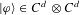. Up to local change of bases, any of these states can be written as17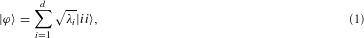where λi are the (real) Schmidt coefficients such that λ1λ2λd≥0. This configuration reminds us of the states underlying the so-called projected pair-entangled states18, and thus we call these networks pair-entangled pure networks. For these geometries, we first introduce a series of protocols that are closely related to classical percolation6, a concept that appears in statistical mechanics. We then determine the optimal protocols for several one-dimensional configurations, where some counterintuitive phenomena occur. We use these phenomena to introduce various protocols in more complex two-dimensional configurations.

We show that these new protocols provide a dramatic improvement over those based on classical percolation, in the sense that we can obtain perfect quantum communication even though the classical percolation protocols give rise to an exponential decay of the success probability with the number of nodes. In fact, we will argue that there exists an entanglement phase transition in the quantum networks, which may be exploited to obtain very efficient protocols. Thus, this work opens a new set of problems in quantum-information theory, which are related to statistical physics, but pose completely new challenges in these fields. As opposed to most of the recent work on entanglement theory, which has been devoted to using some of the tools developed so far in quantum-information theory to analyse problems in statistical mechanics18,19,20,21, the present work takes a step in the converse direction.

Let us start by considering a natural measurement consisting of all the pairs of nodes locally transforming their states into singlets with optimal probability pok. Recall that the SCP for a state (1) is known to be equal to pok=min(1,2(1−λ1)) (ref. 22). Then, a perfect quantum channel between the nodes is established with probability pok, otherwise no entanglement is left. This problem is equivalent to a standard bond-percolation situation6, where one distributes connections among the nodes of a lattice in a probabilistic way: with probability p an edge connecting a pair of nodes is established, otherwise the nodes are kept unconnected. We call this measurement strategy classical entanglement percolation (CEP). In bond percolation, for each lattice geometry there exists a percolation threshold probability, pth, such that an infinite connected cluster can be established if and only if p>pth (see also Table 1). The probability θ(p) that a given node belongs to an infinite cluster, or percolation probability, is strictly positive for p>pth, and zero otherwise (in the limit of an infinite number of nodes). Then, the probability that two given distant nodes can be connected by a path is distance independent and is given, correspondingly, by θ2(p) for p>pth; for ppth this probability decays exponentially with the number of nodes, N, separating the two distant ones.

The threshold probabilities define a minimal amount of entanglement for the initial state such that CEP is possible. In the case of one-dimensional chains (see Fig. 2) percolation is possible if and only if p=1. Therefore, the SCP decays exponentially with N unless the states are more entangled than the singlet, in the sense that pok=1. In a square two-dimensional lattice, the entanglement threshold derived from percolation arguments (see Table 1) is pok=2(1−λ1)=1/2.

The CEP strategy already shows that the distribution of entanglement through quantum networks defines a critical phenomenon. For instance, for two-dimensional lattices and qubits, there exists a continuous interval for λ2 such that the probability of having an infinitely connected entangled path is unity, whereas for product states, that is when λ2=0, this probability is zero. Because there is no analytic function fulfilling these requirements, the probability of infinite distance entanglement must be non-analytic, indicating a sharp transition.

Now, it is natural to wonder whether CEP is optimal for any geometry and number of nodes, and if not to see whether, at least, it predicts the correct decay of entanglement in the asymptotic limit. Next, we show that, for one-dimensional chains, although CEP is not optimal for some finite N, it gives the right asymptotic behaviour. Moving to two-dimensional networks, we prove that CEP is not optimal even in the asymptotic case. Thus, the problem of entanglement distribution through quantum networks defines a new type of critical phenomenon, with new threshold values, which we call entanglement percolation.

The scenario of a one-dimensional chain configuration (see Fig. 2) consists of two end nodes connected by several repeaters10. As said, all the bonds are equal to |ϕ〉. We start out with the case of qubits, d=2. A surprising result already appears in the first non-trivial situation consisting of one repeater. An upper bound to the SCP in the one-repeater scenario is obtained by putting nodes A and R1 together, which implies that the SCP cannot be larger than pok. This bound can indeed be achieved by means of a rather simple protocol involving entanglement swapping23 at the repeater. However, if CEP is applied, the obtained SCP is simply (pok)2. This proves that CEP is not optimal already for the one-repeater configuration. We find it rather counter-intuitive that the intermediate repeater does not decrease the optimal SCP. This behaviour, however, does not survive in the asymptotic limit. In this limit the so-called concurrence24, another measure of entanglement, decreases exponentially with the number of repeaters, unless the connecting states are maximally entangled (see the Methods section). The exponential decay of the SCP automatically follows.

Most of these results can be generalized to higher-dimensional systems, d>2. For the one-repeater configuration, the SCP is again equal to pok. It suffices to map the initial state into a two-qubit state, without changing the SCP, and then apply the previous protocol. Moving to the asymptotic limit, an exponential decay of the SCP with N can be proven in the scenario where the measurement strategies involve only one-way communication. First, a measurement is made at the first repeater. The result is communicated to the second repeater, where a second measurement is applied. The results of the two measurements are communicated to the third, and so on until the last repeater, where the final measurement depends on all the previous results.

Putting all these results together, a unified picture emerges for the distribution of entanglement in one-dimensional chains: despite some remarkable effects for finite N, the SCP decreases exponentially with the number of repeaters whenever the connecting bonds have less entanglement than a singlet. The CEP strategy fails for some finite configurations, but predicts the correct behaviour in the asymptotic limit.

The situation becomes much richer for two-dimensional geometries. First, we consider finite two-dimensional lattices. The non-optimality of CEP can be shown already for the simplest 2×2 square lattice and qubits. Consider the two non-neighbouring sites in the main diagonal of the square. The SCP obtained by CEP is 1−(1−(pok)2)2. By concatenating the optimal measurement strategy for the one-repeater configuration, the SCP is 1−(1−pok)2. However none of these strategies exploits the richness of the two-dimensional configuration. Indeed, we can design strategies such that a singlet can be established with unit probability whenever |ϕ〉 satisfies 1/2≤λ1≤0.6498. Thus, there are two-dimensional network geometries where, although the connections are not maximally entangled, the entanglement is still sufficient to establish a perfect quantum channel.

Let us now see whether the thresholds defined by standard percolation theory are optimal for asymptotically large networks. In the next lines, we construct an example that goes beyond the classical percolation picture, proving that the CEP strategy is not optimal. The key ingredient for this construction is the measurement derived above for the one-repeater configuration, which gave rise to an SCP equal to pok. Our example considers a honeycomb lattice where each node is connected by two copies of the same two-qubit state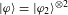(see Fig. 3a). If, as above, the Schmidt coefficients of the two-qubit state are λ1λ2, the SCP of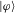is given by pok=2(1−λ12). We choose this conversion probability smaller than the percolation threshold for the honeycomb lattice, which gives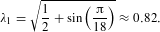Therefore, CEP is useless. Now, half of the nodes carry out the optimal strategy for the one-repeater configuration, mapping the honeycomb lattice into a triangular lattice, as shown in Fig. 3. The SCP for the new bonds is exactly the same as for the state |ϕ2〉, that is 2λ2. This probability is larger than the percolation threshold for the triangular lattice, because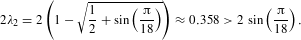The nodes can now apply CEP to the new lattice and succeed. Thus, this strategy, which combines entanglement swapping and CEP, allows us to establish a perfect quantum channel in a network where CEP fails.Figure 3: Example of a quantum network where entanglement percolation and CEP are not equivalent.

Two comments are relevant at this point. First, although the previous strategy uses classical percolation concepts, this is not necessarily the case for the optimal (unknown) strategy, which may exploit different techniques. Second, our results do not exclude the possibility that for two-dimensional lattices the critical amount of entanglement is zero. The bound for the entanglement phase transition would then be somewhat trivial, but the implications from a quantum-communication viewpoint would be very relevant: any amount of entanglement between the nodes would be sufficient for entanglement percolation.

We have shown that the distribution of entanglement through quantum networks defines a framework where statistical methods and concepts, such as classical percolation theory and beyond, naturally apply. It leads to a novel type of critical phenomenon, an entanglement phase transition that we call entanglement percolation. The corresponding critical parameter is the minimal amount of entanglement necessary to establish a perfect distant quantum channel with significant (non-exponentially decaying) probability. Further understanding of optimal entanglement-percolation strategies is necessary for the future development and prosperity of quantum networks.

## Methods

### One-dimensional chains

We start by showing that the concurrence decays exponentially with the number of nodes in a one-dimensional chain of qubits when the connecting states are not maximally entangled. Recall that, given a two-qubit pure state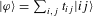, its concurrence reads C(ϕ)=2|det(T)|, where T is the 2×2 matrix such that (T)i j=ti j.

When considering the repeater configuration, the maximization of the averaged concurrence turns out to be equal to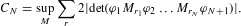Here M briefly denotes the choice of measurements, whereas ϕk represent the 2×2 diagonal matrices given by the Schmidt coefficients of the states |ϕk〉. EquationSource math mrow msub miM mrow msub mir mik are also 2×2 matrices, corresponding to the pure state |rk〉 associated with the measurement result rk of the kth repeater, that is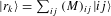. Note that the computational bases i and j in the previous expressions are the Schmidt bases for the states |ϕk〉 and |ϕk+1〉 entering the repeater k. Using the fact that det(A B)=det(A)det(B), the previous maximization gives25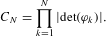Note that 2|det(ϕk)|=1 if and only if |ϕk〉 is maximally entangled, which proves the announced result.

Most of the results derived in the qubit case can be generalized to arbitrary dimension. Let us first consider the one-repeater configuration. Given a state |ϕk〉, see equation (1), it is always possible to transform this state in a deterministic way into a two-qubit state of Schmidt coefficients (λ1,1−λ1) by local operations and classical communication. This follows from the application of majorization theory to the study of local operations and classical communication transformations between entangled states26. Note that the SCP for the two states is the same, pok=min(1,2(1−λ1)).

In the case of arbitrary N, an exponential decay for the qubit concurrence can be shown for protocols with one-way communication. Given an arbitrary chain, we consider the almost identical chain where the first state is replaced by a two-qubit entangled state. It is relatively easy to prove that the SCP decays exponentially in the first chain if and only if it does it in the second one. We start with the simplest one-repeater configuration. The quantity to be optimized reads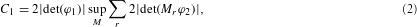where, as above, ϕ1 (ϕ2) is the 2×2 (d×d) matrix corresponding to |ϕ1〉 (|ϕ2〉), whereas Mr is a 2×d matrix associated with the measurement outcome r at the repeater. Thus, we recognize in the r.h.s. of equation (2) the optimal average concurrence we can obtain out of |ϕ2〉 by measurements on one particle that correspond to operators of rank 2. We denote this quantity by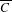, the Schmidt coefficients corresponding to |ϕ2〉 by λ1λ2λd and its SCP by pok, as above. For the outcome r, which occurs with probability pr, μ1rμ2r denotes the Schmidt coefficients corresponding to the resulting two-qubit state |ϕr〉. With this notation, we have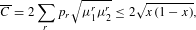where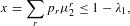and the last inequality follows from the majorization criterion26. The optimal value is obtained for x=pok/2, which is achieved when p1=1 and saturates the two previous inequalities. Thus, we obtain the equalityNote that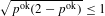, with equality if and only if pok=1. Note also, and this is important for what follows, that the optimal strategy depends only on |ϕ2〉, and not on the first two-qubit state, |ϕ1〉.

This strategy can be generalized to the case of N repeaters when the measurements proceed from left to right. We show this generalization for the case N=2; the case of arbitrary N will immediately follow. Consider the measurement step in the second repeater. After receiving the information about the measurement result in the first repeater, r1, R2 has to measure its particles. For each value of r1, and because A is a qubit, A and R2 share a two-qubit pure state, EquationSource math mrow mo| msub miφ mrow msub mir mn1 mo〉 . Therefore, for each measurement result, R2 is back at the previous one-repeater situation. The optimal measurement strategy in this case was independent of the entanglement of the first two-qubit state. Thus, up to local unitary transformations, the measurement to be applied in the second repeater is independent of r1, and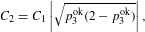where pkok is defined as above for the state |ϕk〉. It is straightforward that this reasoning generalizes to an arbitrary number of repeaters, so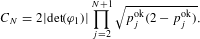Therefore, the average concurrence decreases exponentially with the number of repeaters unless the connecting pure states have pok=1. A non-exponential decay of the SCP when pok<1 would contradict this result.

## References

1. Cirac, J. I., Zoller, P., Kimble, H. J. & Mabuchi, H. Quantum state transfer and entanglement distribution among distant nodes in a quantum network. Phys. Rev. Lett. 78, 3221–3224 (1997).

2. Bennett, C. H. & Brassard, G. in Proc. Int. Conf. on Computer Systems and Signal Processing, Bangalore 175–179 (IEEE, New York, 1984).

3. Ekert, A. K. Quantum cryptography based on Bell’s theorem. Phys. Rev. Lett. 67, 661–664 (1991).

4. Buhrman, H., Cleve, R., Watrous, J. & de Wolf, R. Quantum fingerprinting. Phys. Rev. Lett. 87, 167902 (2001).

5. Bennett, C. H. et al. Teleporting an unknown quantum state via dual classical and Einstein–Podolski–Rosen channels. Phys. Rev. Lett. 70, 1895–1898 (1993).

6. Grimmett, G. Percolation (Springer, Berlin, 1999).

7. Sachdev, S. Quantum Phase Transitions (Cambridge Univ. Press, Cambridge, 1999).

8. Knill, E., Laflamme, R. & Milburn, G. J. A scheme for efficient quantum computation with linear optics. Nature 409, 46–52 (2001).

9. Duan, L. M., Lukin, M. D., Cirac, J. I. & Zoller, P. Long-distance quantum communication with atomic ensembles and linear optics. Nature 414, 413–418 (2001).

10. Briegel, H. J., Dür, W., Cirac, J. I. & Zoller, P. Quantum repeaters: The role of imperfect local operations in quantum communication. Phys. Rev. Lett. 81, 5932–5935 (1998).

11. Chanelière, T. et al. Storage and retrieval of single photons transmitted between remote quantum memories. Nature 438, 833–836 (2005).

12. Tanzilli, S. et al. A photonic quantum information interface. Nature 437, 116–120 (2005).

13. Törmä, P. Transitions in quantum networks. Phys. Rev. Lett. 81, 2185–2189 (1998).

14. Leung, D., Oppenheim, J. & Winter, A. Quantum network communication—the butterfly and beyond. Preprint at &lt;http://arxiv.org/abs/quant-ph/0608223&gt; (2006).

15. Verstraete, F., Popp, M. & Cirac, J. I. Entanglement versus correlations in spin systems. Phys. Rev. Lett. 92, 027901 (2004).

16. Popp, M., Verstraete, F., Martín-Delgado, M. A. & Cirac, J. I. Localizable entanglement. Phys. Rev. A 71, 042306 (2005).

17. Nielsen, M. A. & Chuang, I. L. Quantum Computation and Quantum Information (Cambridge Univ. Press, Cambridge, 2000).

18. Verstraete, F. & Cirac, J. I. Renormalization algorithms for quantum-many body systems in two and higher dimensions. Preprint at &lt;http://arxiv.org/abs/cond-mat/0407066&gt; (2004).

19. Osterloh, A., Amico, L., Falci, G. & Fazio, R. Scaling of entanglement close to a quantum phase transition. Nature 416, 608–610 (2002).

20. Osborne, T. J. & Nielsen, M. A. Entanglement in a simple quantum phase transition. Phys. Rev. A 66, 032110 (2002).

21. Vidal, G., Latorre, J. I., Rico, E. & Kitaev, A. Entanglement in quantum critical phenomena. Phys. Rev. Lett. 90, 227902 (2003).

22. Vidal, G. Entanglement of pure states for a single copy. Phys. Rev. Lett. 83, 1046–1049 (1999).

23. Żukowski, M., Zeilinger, A., Horne, M. A. & Ekert, A. K. Event-ready detectors Bell experiment via entanglement swapping. Phys. Rev. Lett. 71, 4287–4290 (1993).

24. Wootters, W. K. Entanglement of formation of an arbitrary state of two qubits. Phys. Rev. Lett. 80, 2245–2248 (1998).

25. Verstraete, F., Martín-Delgado, M. A. & Cirac, J. I. Diverging entanglement length in gapped quantum spin systems. Phys. Rev. Lett. 92, 087201 (2004).

26. Nielsen, M. A. Conditions for a class of entanglement transformations. Phys. Rev. Lett. 83, 436–439 (1999).

## Acknowledgements

We thank F. Verstraete, J. Wehr and M. M. Wolf for discussion. We acknowledge support from Deutsche Forschungsgemeinschaft, EU IP Programmes ‘SCALA’ and ‘QAP’, European Science Foundation PESC QUDEDIS, MEC (Spanish Government) under contracts FIS 2005-04627, FIS 2004-05639, ‘Ramón y Cajal’ and Consolider QOIT.

## Author information

Authors

### Corresponding author

Correspondence to J. Ignacio Cirac.

## Ethics declarations

### Competing interests

The authors declare no competing financial interests.

## Rights and permissions

Reprints and Permissions

Acín, A., Cirac, J. & Lewenstein, M. Entanglement percolation in quantum networks. Nature Phys 3, 256–259 (2007). https://doi.org/10.1038/nphys549

• Accepted:

• Published:

• Issue Date:

• DOI: https://doi.org/10.1038/nphys549

• ### Entanglement generation in a quantum network at distance-independent rate

• Ashlesha Patil
• Mihir Pant
• Saikat Guha

npj Quantum Information (2022)

• ### Entanglement formation in continuous-variable random quantum networks

• Bingzhi Zhang
• Quntao Zhuang

npj Quantum Information (2021)

• ### Effective routing design for remote entanglement generation on quantum networks

• Changhao Li
• Tianyi Li
• Paola Cappellaro

npj Quantum Information (2021)

• ### A quantum network node with crossed optical fibre cavities

• Manuel Brekenfeld
• Dominik Niemietz
• Gerhard Rempe

Nature Physics (2020)

• ### Percolation transition control in quantum networks

• Michael Siomau

Quantum Information Processing (2020)

## Search